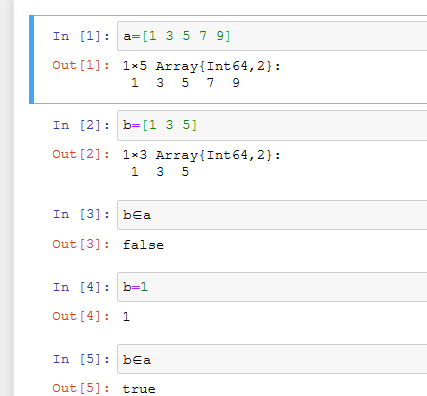# Julia的 in 为什么不对？1 个赞
a = [1, 2, 3, 4, 5]
b = [1, 2, 3]
b in a


findall(x->x in [1, 3, 5], [1, 4, 5, 7, 9])


1 个赞

\in 表示 属于
\subset 表示子集

1 个赞

help?> issubset
search: issubset

issubset(a, b)
⊆(a,b)  -> Bool
⊇(b, a) -> Bool

Determine whether every element of a is also in b, using in.

Examples
≡≡≡≡≡≡≡≡≡≡

julia> issubset([1, 2], [1, 2, 3])
true

julia> [1, 2, 3] ⊆ [1, 2]
false

julia> [1, 2, 3] ⊇ [1, 2]
true


julia> a=[1 3 5 7 9]
1×5 Array{Int64,2}:
1  3  5  7  9

julia> b=[1 3 5]
1×3 Array{Int64,2}:
1  3  5

julia> b ⊆ a
true

julia> a = [1 3 5 7 9]
1×5 Array{Int64,2}:
1  3  5  7  9

julia> b = [1 3 5]
1×3 Array{Int64,2}:
1  3  5

julia> indexin(b, a)
1×3 Array{Union{Nothing, CartesianIndex{2}},2}:
CartesianIndex(1, 1)  CartesianIndex(1, 2)  CartesianIndex(1, 3)


julia> a = [1,3,5,7,9]
5-element Array{Int64,1}:
1
3
5
7
9

julia> b = [1,3,5]
3-element Array{Int64,1}:
1
3
5

julia> indexin(b, a)
3-element Array{Union{Nothing, Int64},1}:
1
2
3

julia> a[indexin(b, a)]
3-element Array{Int64,1}:
1
3
5


2 个赞